This notebook contains an excerpt from the Python Data Science Handbook by Jake VanderPlas; the content is available on GitHub.

The text is released under the CC-BY-NC-ND license, and code is released under the MIT license. If you find this content useful, please consider supporting the work by buying the book!

No changes were made to the contents of this notebook from the original.

# Multiple Subplots¶

Sometimes it is helpful to compare different views of data side by side. To this end, Matplotlib has the concept of subplots: groups of smaller axes that can exist together within a single figure. These subplots might be insets, grids of plots, or other more complicated layouts. In this section we'll explore four routines for creating subplots in Matplotlib.

In :
%matplotlib inline
import matplotlib.pyplot as plt
plt.style.use('seaborn-white')
import numpy as np


## plt.axes: Subplots by Hand¶

The most basic method of creating an axes is to use the plt.axes function. As we've seen previously, by default this creates a standard axes object that fills the entire figure. plt.axes also takes an optional argument that is a list of four numbers in the figure coordinate system. These numbers represent [left, bottom, width, height] in the figure coordinate system, which ranges from 0 at the bottom left of the figure to 1 at the top right of the figure.

For example, we might create an inset axes at the top-right corner of another axes by setting the x and y position to 0.65 (that is, starting at 65% of the width and 65% of the height of the figure) and the x and y extents to 0.2 (that is, the size of the axes is 20% of the width and 20% of the height of the figure):

In :
ax1 = plt.axes()  # standard axes
ax2 = plt.axes([0.65, 0.65, 0.2, 0.2])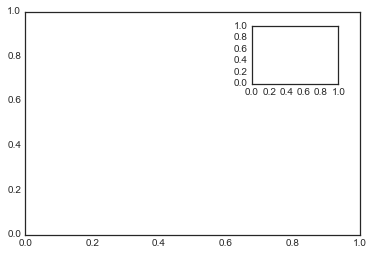The equivalent of this command within the object-oriented interface is fig.add_axes(). Let's use this to create two vertically stacked axes:

In :
fig = plt.figure()
ax1 = fig.add_axes([0.1, 0.5, 0.8, 0.4],
xticklabels=[], ylim=(-1.2, 1.2))
ax2 = fig.add_axes([0.1, 0.1, 0.8, 0.4],
ylim=(-1.2, 1.2))

x = np.linspace(0, 10)
ax1.plot(np.sin(x))
ax2.plot(np.cos(x));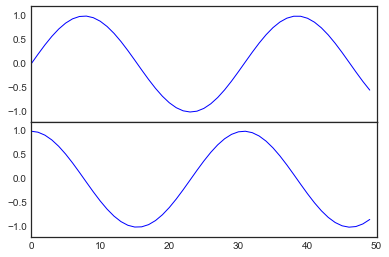We now have two axes (the top with no tick labels) that are just touching: the bottom of the upper panel (at position 0.5) matches the top of the lower panel (at position 0.1 + 0.4).

## plt.subplot: Simple Grids of Subplots¶

Aligned columns or rows of subplots are a common-enough need that Matplotlib has several convenience routines that make them easy to create. The lowest level of these is plt.subplot(), which creates a single subplot within a grid. As you can see, this command takes three integer arguments—the number of rows, the number of columns, and the index of the plot to be created in this scheme, which runs from the upper left to the bottom right:

In :
for i in range(1, 7):
plt.subplot(2, 3, i)
plt.text(0.5, 0.5, str((2, 3, i)),
fontsize=18, ha='center')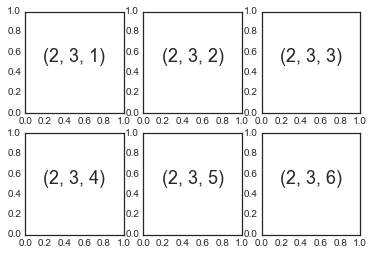The command plt.subplots_adjust can be used to adjust the spacing between these plots. The following code uses the equivalent object-oriented command, fig.add_subplot():

In :
fig = plt.figure()
for i in range(1, 7):
ax.text(0.5, 0.5, str((2, 3, i)),
fontsize=18, ha='center')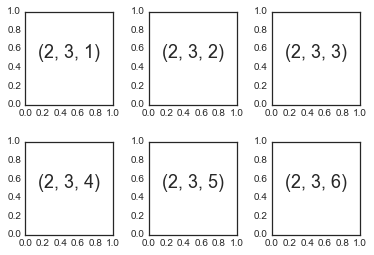We've used the hspace and wspace arguments of plt.subplots_adjust, which specify the spacing along the height and width of the figure, in units of the subplot size (in this case, the space is 40% of the subplot width and height).

## plt.subplots: The Whole Grid in One Go¶

The approach just described can become quite tedious when creating a large grid of subplots, especially if you'd like to hide the x- and y-axis labels on the inner plots. For this purpose, plt.subplots() is the easier tool to use (note the s at the end of subplots). Rather than creating a single subplot, this function creates a full grid of subplots in a single line, returning them in a NumPy array. The arguments are the number of rows and number of columns, along with optional keywords sharex and sharey, which allow you to specify the relationships between different axes.

Here we'll create a $2 \times 3$ grid of subplots, where all axes in the same row share their y-axis scale, and all axes in the same column share their x-axis scale:

In :
fig, ax = plt.subplots(2, 3, sharex='col', sharey='row')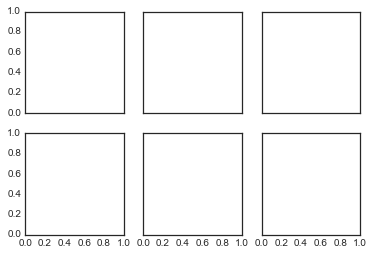Note that by specifying sharex and sharey, we've automatically removed inner labels on the grid to make the plot cleaner. The resulting grid of axes instances is returned within a NumPy array, allowing for convenient specification of the desired axes using standard array indexing notation:

In :
# axes are in a two-dimensional array, indexed by [row, col]
for i in range(2):
for j in range(3):
ax[i, j].text(0.5, 0.5, str((i, j)),
fontsize=18, ha='center')
fig

Out: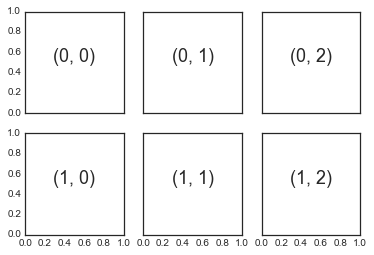In comparison to plt.subplot(), plt.subplots() is more consistent with Python's conventional 0-based indexing.

## plt.GridSpec: More Complicated Arrangements¶

To go beyond a regular grid to subplots that span multiple rows and columns, plt.GridSpec() is the best tool. The plt.GridSpec() object does not create a plot by itself; it is simply a convenient interface that is recognized by the plt.subplot() command. For example, a gridspec for a grid of two rows and three columns with some specified width and height space looks like this:

In :
grid = plt.GridSpec(2, 3, wspace=0.4, hspace=0.3)


From this we can specify subplot locations and extents using the familiary Python slicing syntax:

In :
plt.subplot(grid[0, 0])
plt.subplot(grid[0, 1:])
plt.subplot(grid[1, :2])
plt.subplot(grid[1, 2]);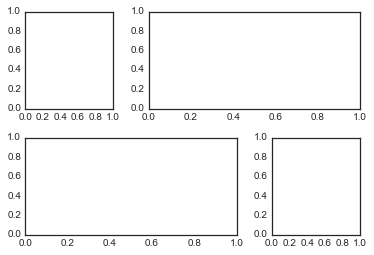This type of flexible grid alignment has a wide range of uses. I most often use it when creating multi-axes histogram plots like the ones shown here:

In :
# Create some normally distributed data
mean = [0, 0]
cov = [[1, 1], [1, 2]]
x, y = np.random.multivariate_normal(mean, cov, 3000).T

# Set up the axes with gridspec
fig = plt.figure(figsize=(6, 6))
grid = plt.GridSpec(4, 4, hspace=0.2, wspace=0.2)
y_hist = fig.add_subplot(grid[:-1, 0], xticklabels=[], sharey=main_ax)
x_hist = fig.add_subplot(grid[-1, 1:], yticklabels=[], sharex=main_ax)

# scatter points on the main axes
main_ax.plot(x, y, 'ok', markersize=3, alpha=0.2)

# histogram on the attached axes
x_hist.hist(x, 40, histtype='stepfilled',
orientation='vertical', color='gray')
x_hist.invert_yaxis()

y_hist.hist(y, 40, histtype='stepfilled',
orientation='horizontal', color='gray')
y_hist.invert_xaxis()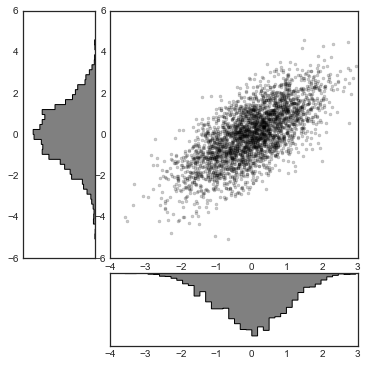This type of distribution plotted alongside its margins is common enough that it has its own plotting API in the Seaborn package; see Visualization With Seaborn for more details.